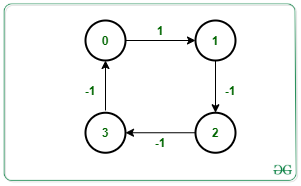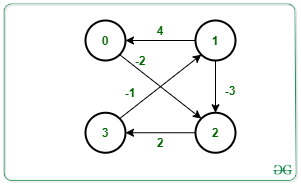Related Articles
Detect a negative cycle in a Graph using Shortest Path Faster Algorithm
• Difficulty Level : Medium
• Last Updated : 22 Oct, 2020

Given a graph G consisting of nodes valued [0, N – 1], a source S, and an array Edges[] of type {u, v, w} that denotes that there is a directed edge between node u and v with weight w, the task is to check if there exists a negative cycle from the given source. If found to be true, then print “Yes”. Otherwise, print “No”.

A negative cycle is a cycle in which the sum of all its weight in that cycle is negative.

Examples:

Input: N = 4, M = 4, Edges[][] = {{0, 1, 1}, {1, 2, -1}, {2, 3, -1}, {3, 0, -1}}, S = 0
Output: Yes
Explanation:Starting from the source node 0, the graph contains cycle as 0 -> 1 -> 2 -> 3 -> 0.
The sum of weight in the above path is 1 – 1 – 1 – 1 = -2.
Therefore, the graph contains a negative cycle.

Input: N = 4, M = 5, Edges[][] = {{0, 2, -2}, {1, 0, 4}, {1, 2, -3}, {2, 3}, {3, 1}}, W[] = {-2, 4, -3, 2, -1}, S = 1
Output: Yes
Explanation:Starting from the source node 1, the graph contains cycle as 1 -> 2 -> 3 -> 1.
The sum of weight in the above path is -3 + 2 – 1 = -2.
Therefore, the graph contains a negative cycle.

Approach: The idea is to use the Shortest Path Faster Algorithm(SPFA) to find if a negative cycle is present and reachable from the source vertex in a graph. Follow the below steps to solve the problem:

• Initialize the arrays dis[] with large value, vis[] with false and cnt[] to store the count about how many times a vertex has been relaxed.
• Traverse the graph using the SPFA algorithm.
• Increment the count for each vertex whenever the vertex is relaxed.

The term relaxation means updating the cost of all vertices connected to a vertex v if those costs would be improved by including the path via vertex v.

• Stop the algorithm and print “Yes” as soon as some vertex got relaxed for the Nth time as there are only N vertices i.e., from 0 to N – 1.
• Otherwise, print “No”.

Below is the implementation of the above approach:

## C++

 `// C++ program for the above approach``#include ``using` `namespace` `std;` `bool` `sfpa(``int` `V, ``int` `src, ``int` `Edges[],``          ``int` `M)``{``    ``// Stores the adjcency list of``    ``// the given graph``    ``vector > g[V];` `    ``// Create Adjacency List``    ``for` `(``int` `i = 0; i < M; i++) {` `        ``int` `u = Edges[i];``        ``int` `v = Edges[i];``        ``int` `w = Edges[i];` `        ``g[u].push_back({ v, w });``    ``}` `    ``// Stores the distance of all``    ``// reachable vertex from source``    ``vector<``int``> dist(V, INT_MAX);` `    ``// Check if vertex is present``    ``// in queue or not``    ``vector<``bool``> inQueue(V, ``false``);` `    ``// Counts the relaxation for``    ``// each vertex``    ``vector<``int``> cnt(V, 0);` `    ``// Distance from src to src is 0``    ``dist[src] = 0;` `    ``// Create a queue``    ``queue<``int``> q;` `    ``// Push source in the queue``    ``q.push(src);` `    ``// Mark source as visited``    ``inQueue[src] = ``true``;` `    ``while` `(!q.empty()) {` `        ``// Front vertex of Queue``        ``int` `u = q.front();``        ``q.pop();` `        ``inQueue[u] = ``false``;` `        ``// Relaxing all edges of``        ``// vertex from the Queue``        ``for` `(pair<``int``, ``int``> x : g[u]) {` `            ``int` `v = x.first;``            ``int` `cost = x.second;` `            ``// Update the dist[v] to``            ``// minimum distance``            ``if` `(dist[v] > dist[u] + cost) {` `                ``dist[v] = dist[u] + cost;` `                ``// If vertex v is in Queue``                ``if` `(!inQueue[v]) {``                    ``q.push(v);``                    ``inQueue[v] = ``true``;``                    ``cnt[v]++;` `                    ``// Negative cycle``                    ``if` `(cnt[v] >= V)``                        ``return` `true``;``                ``}``            ``}``        ``}``    ``}` `    ``// No cycle found``    ``return` `false``;``}` `// Driver Code``int` `main()``{``    ``// Number of vertices``    ``int` `N = 4;` `    ``// Given source node src``    ``int` `src = 0;` `    ``// Number of Edges``    ``int` `M = 4;` `    ``// Given Edges with weight``    ``int` `Edges[] = { { 0, 1, 1 },``                       ``{ 1, 2, -1 },``                       ``{ 2, 3, -1 },``                       ``{ 3, 0, -1 } };` `    ``// If cycle is present``    ``if` `(sfpa(N, src, Edges, M) == ``true``)``        ``cout << ``"Yes"` `<< endl;``    ``else``        ``cout << ``"No"` `<< endl;` `    ``return` `0;``}`

## Java

 `// Java program for``// the above approach``import` `java.util.*;``class` `GFG{``    ` `static` `class` `pair``{``  ``int` `first, second;``  ``public` `pair(``int` `first,``              ``int` `second) ``  ``{``    ``this``.first = first;``    ``this``.second = second;``  ``}   ``}``  ` `static` `boolean` `sfpa(``int` `V, ``int` `src,``                    ``int` `Edges[][], ``int` `M)``{``  ``// Stores the adjcency list of``  ``// the given graph``  ``Vector []g = ``new` `Vector[V];``  ``for` `(``int` `i = ``0``; i < V; i++)``  ``{``    ``g[i] = ``new` `Vector();``  ``}` `  ``// Create Adjacency List``  ``for` `(``int` `i = ``0``; i < M; i++)``  ``{``    ``int` `u = Edges[i][``0``];``    ``int` `v = Edges[i][``1``];``    ``int` `w = Edges[i][``2``];``    ``g[u].add(``new` `pair(v, w));``  ``}` `  ``// Stores the distance of all``  ``// reachable vertex from source``  ``int` `[]dist = ``new` `int``[V];``  ``Arrays.fill(dist, Integer.MAX_VALUE);` `  ``// Check if vertex is present``  ``// in queue or not``  ``boolean` `[]inQueue = ``new` `boolean``[V];` `  ``// Counts the relaxation for``  ``// each vertex``  ``int` `[]cnt = ``new` `int``[V];` `  ``// Distance from src``  ``// to src is 0``  ``dist[src] = ``0``;` `  ``// Create a queue``  ``Queue q = ``new` `LinkedList<>();` `  ``// Push source in the queue``  ``q.add(src);` `  ``// Mark source as visited``  ``inQueue[src] = ``true``;` `  ``while` `(!q.isEmpty())``  ``{``    ``// Front vertex of Queue``    ``int` `u = q.peek();``    ``q.remove();` `    ``inQueue[u] = ``false``;` `    ``// Relaxing all edges of``    ``// vertex from the Queue``    ``for` `(pair x : g[u])``    ``{``      ``int` `v = x.first;``      ``int` `cost = x.second;` `      ``// Update the dist[v] to``      ``// minimum distance``      ``if` `(dist[v] > dist[u] + cost)``      ``{``        ``dist[v] = dist[u] + cost;` `        ``// If vertex v is in Queue``        ``if` `(!inQueue[v])``        ``{``          ``q.add(v);``          ``inQueue[v] = ``true``;``          ``cnt[v]++;` `          ``// Negative cycle``          ``if` `(cnt[v] >= V)``            ``return` `true``;``        ``}``      ``}``    ``}``  ``}` `  ``// No cycle found``  ``return` `false``;``}` `// Driver Code``public` `static` `void` `main(String[] args)``{``  ``// Number of vertices``  ``int` `N = ``4``;` `  ``// Given source node src``  ``int` `src = ``0``;` `  ``// Number of Edges``  ``int` `M = ``4``;` `  ``// Given Edges with weight``  ``int` `Edges[][] = {{``0``, ``1``, ``1``},``                   ``{``1``, ``2``, -``1``},``                   ``{``2``, ``3``, -``1``},``                   ``{``3``, ``0``, -``1``}};` `  ``// If cycle is present``  ``if` `(sfpa(N, src, Edges, M) == ``true``)``    ``System.out.print(``"Yes"` `+ ``"\n"``);``  ``else``    ``System.out.print(``"No"` `+ ``"\n"``);``}``}` `// This code is contributed by 29AjayKumar`

## Python3

 `# Python3 program for the above approach``import` `sys` `def` `sfpa(V, src, Edges, M):``    ` `    ``# Stores the adjcency list of``    ``# the given graph``    ``g ``=` `[[] ``for` `i ``in` `range``(V)]` `    ``# Create Adjacency List``    ``for` `i ``in` `range``(M):``        ``u ``=` `Edges[i][``0``]``        ``v ``=` `Edges[i][``1``]``        ``w ``=` `Edges[i][``2``]` `        ``g[u].append([v, w])` `    ``# Stores the distance of all``    ``# reachable vertex from source``    ``dist ``=` `[sys.maxsize ``for` `i ``in` `range``(V)]` `    ``# Check if vertex is present``    ``# in queue or not``    ``inQueue ``=` `[``False` `for` `i ``in` `range``(V)]` `    ``# Counts the relaxation for``    ``# each vertex``    ``cnt ``=` `[``0` `for` `i ``in` `range``(V)]` `    ``# Distance from src to src is 0``    ``dist[src] ``=` `0` `    ``# Create a queue``    ``q ``=` `[]` `    ``# Push source in the queue``    ``q.append(src)` `    ``# Mark source as visited``    ``inQueue[src] ``=` `True` `    ``while` `(``len``(q)):``        ` `        ``# Front vertex of Queue``        ``u ``=` `q[``0``]``        ``q.remove(q[``0``])` `        ``inQueue[u] ``=` `False` `        ``# Relaxing all edges of``        ``# vertex from the Queue``        ``for` `x ``in` `g[u]:``            ``v ``=` `x[``0``]``            ``cost ``=` `x[``1``]` `            ``# Update the dist[v] to``            ``# minimum distance``            ``if` `(dist[v] > dist[u] ``+` `cost):``                ``dist[v] ``=` `dist[u] ``+` `cost` `                ``# If vertex v is in Queue``                ``if` `(inQueue[v] ``=``=` `False``):``                    ``q.append(v)``                    ``inQueue[v] ``=` `True``                    ``cnt[v] ``+``=` `1` `                    ``# Negative cycle``                    ``if` `(cnt[v] >``=` `V):``                        ``return` `True` `    ``# No cycle found``    ``return` `False` `# Driver Code``if` `__name__ ``=``=` `'__main__'``:``    ` `    ``# Number of vertices``    ``N ``=` `4` `    ``# Given source node src``    ``src ``=` `0` `    ``# Number of Edges``    ``M ``=` `4` `    ``# Given Edges with weight``    ``Edges ``=` `[ [ ``0``, ``1``, ``1` `],``              ``[ ``1``, ``2``, ``-``1` `],``              ``[ ``2``, ``3``, ``-``1` `],``              ``[ ``3``, ``0``, ``-``1` `] ]` `    ``# If cycle is present``    ``if` `(sfpa(N, src, Edges, M) ``=``=` `True``):``        ``print``(``"Yes"``)``    ``else``:``        ``print``(``"No"``)` `# This code is contributed by SURENDRA_GANGWAR`

## C#

 `// C# program for``// the above approach``using` `System;``using` `System.Collections.Generic;``class` `GFG{``    ` `class` `pair``{``  ``public` `int` `first, second;``  ``public` `pair(``int` `first,``              ``int` `second) ``  ``{``    ``this``.first = first;``    ``this``.second = second;``  ``}   ``}``  ` `static` `bool` `sfpa(``int` `V, ``int` `src,``                 ``int` `[,]Edges, ``int` `M)``{``  ``// Stores the adjcency list of``  ``// the given graph``  ``List []g = ``new` `List[V];``  ``for` `(``int` `i = 0; i < V; i++)``  ``{``    ``g[i] = ``new` `List();``  ``}` `  ``// Create Adjacency List``  ``for` `(``int` `i = 0; i < M; i++)``  ``{``    ``int` `u = Edges[i, 0];``    ``int` `v = Edges[i, 1];``    ``int` `w = Edges[i, 2];``    ``g[u].Add(``new` `pair(v, w));``  ``}` `  ``// Stores the distance of all``  ``// reachable vertex from source``  ``int` `[]dist = ``new` `int``[V];``  ``for` `(``int` `i = 0; i < V; i++)``    ``dist[i] = ``int``.MaxValue;` `  ``// Check if vertex is present``  ``// in queue or not``  ``bool` `[]inQueue = ``new` `bool``[V];` `  ``// Counts the relaxation for``  ``// each vertex``  ``int` `[]cnt = ``new` `int``[V];` `  ``// Distance from src``  ``// to src is 0``  ``dist[src] = 0;` `  ``// Create a queue``  ``Queue<``int``> q = ``new` `Queue<``int``>();` `  ``// Push source in the queue``  ``q.Enqueue(src);` `  ``// Mark source as visited``  ``inQueue[src] = ``true``;` `  ``while` `(q.Count != 0)``  ``{``    ``// Front vertex of Queue``    ``int` `u = q.Peek();``    ``q.Dequeue();` `    ``inQueue[u] = ``false``;` `    ``// Relaxing all edges of``    ``// vertex from the Queue``    ``foreach` `(pair x ``in` `g[u])``    ``{``      ``int` `v = x.first;``      ``int` `cost = x.second;` `      ``// Update the dist[v] to``      ``// minimum distance``      ``if` `(dist[v] > dist[u] + cost)``      ``{``        ``dist[v] = dist[u] + cost;` `        ``// If vertex v is in Queue``        ``if` `(!inQueue[v])``        ``{``          ``q.Enqueue(v);``          ``inQueue[v] = ``true``;``          ``cnt[v]++;` `          ``// Negative cycle``          ``if` `(cnt[v] >= V)``            ``return` `true``;``        ``}``      ``}``    ``}``  ``}` `  ``// No cycle found``  ``return` `false``;``}` `// Driver Code``public` `static` `void` `Main(String[] args)``{``  ``// Number of vertices``  ``int` `N = 4;` `  ``// Given source node src``  ``int` `src = 0;` `  ``// Number of Edges``  ``int` `M = 4;` `  ``// Given Edges with weight``  ``int` `[,]Edges = {{0, 1, 1},``                  ``{1, 2, -1},``                  ``{2, 3, -1},``                  ``{3, 0, -1}};` `  ``// If cycle is present``  ``if` `(sfpa(N, src, Edges, M) == ``true``)``    ``Console.Write(``"Yes"` `+ ``"\n"``);``  ``else``    ``Console.Write(``"No"` `+ ``"\n"``);``}``}` `// This code is contributed by 29AjayKumar`
Output:
```Yes

```

Time Complexity: O(N*M), where N is the number of vertices and M is the number of edges.
Auxiliary Space: O(N + M)

Attention reader! Don’t stop learning now. Get hold of all the important DSA concepts with the DSA Self Paced Course at a student-friendly price and become industry ready.

My Personal Notes arrow_drop_up